# Mean State

Period Mean (original grids) [Watt m-2]
Model Period Mean (intersection) [Watt m-2]
Model Period Mean (complement) [Watt m-2]
Benchmark Period Mean (intersection) [Watt m-2]
Benchmark Period Mean (complement) [Watt m-2]
Bias [Watt m-2]
RMSE [Watt m-2]
Phase Shift [months]
Bias Score 
RMSE Score 
Seasonal Cycle Score 
Spatial Distribution Score 
Interannual Variability Score 
Overall Score 
Benchmark [-] 179.
CLM4 [-] 191. 191. 0.00 177. 216. 13.6 24.0 1.16 0.45 0.45 0.84 0.93 0.51 0.60
CLM4.5 [-] 191. 191. 0.00 177. 216. 13.9 24.3 1.14 0.44 0.45 0.85 0.95 0.52 0.61
CLM5 [-] 191. 191. 0.00 177. 216. 14.0 24.2 1.11 0.44 0.45 0.85 0.95 0.52 0.61
Period Mean (original grids) [Watt m-2]
Model Period Mean (intersection) [Watt m-2]
Model Period Mean (complement) [Watt m-2]
Benchmark Period Mean (intersection) [Watt m-2]
Benchmark Period Mean (complement) [Watt m-2]
Bias [Watt m-2]
RMSE [Watt m-2]
Phase Shift [months]
Bias Score 
RMSE Score 
Seasonal Cycle Score 
Spatial Distribution Score 
Interannual Variability Score 
Overall Score 
Benchmark [-] 189.
CLM4 [-] 196. 196. 0.00 188. 224. 8.04 22.1 1.17 0.59 0.47 0.85 0.89 0.67 0.66
CLM4.5 [-] 197. 197. 0.00 188. 224. 8.98 22.6 1.12 0.58 0.46 0.86 0.89 0.68 0.66
CLM5 [-] 198. 198. 0.00 188. 224. 9.50 22.8 1.64 0.58 0.46 0.76 0.90 0.67 0.64
Period Mean (original grids) [Watt m-2]
Model Period Mean (intersection) [Watt m-2]
Model Period Mean (complement) [Watt m-2]
Benchmark Period Mean (intersection) [Watt m-2]
Benchmark Period Mean (complement) [Watt m-2]
Bias [Watt m-2]
RMSE [Watt m-2]
Phase Shift [months]
Bias Score 
RMSE Score 
Seasonal Cycle Score 
Spatial Distribution Score 
Interannual Variability Score 
Overall Score 
Benchmark [-] 194.
CLM4 [-] 194. 194. 0.00 192. 221. 1.54 22.7 1.16 0.70 0.50 0.84 0.74 0.53 0.63
CLM4.5 [-] 194. 194. 0.00 192. 221. 2.04 22.9 1.19 0.69 0.50 0.84 0.73 0.54 0.63
CLM5 [-] 194. 194. 0.00 192. 221. 2.03 22.6 1.19 0.69 0.50 0.84 0.75 0.53 0.64
Period Mean (original grids) [Watt m-2]
Model Period Mean (intersection) [Watt m-2]
Model Period Mean (complement) [Watt m-2]
Benchmark Period Mean (intersection) [Watt m-2]
Benchmark Period Mean (complement) [Watt m-2]
Bias [Watt m-2]
RMSE [Watt m-2]
Phase Shift [months]
Bias Score 
RMSE Score 
Seasonal Cycle Score 
Spatial Distribution Score 
Interannual Variability Score 
Overall Score 
Benchmark [-] 81.3
CLM4 [-] 66.2 66.4 0.00 80.9 86.2 -15.0 29.1 0.534 0.80 0.68 0.96 0.96 0.69 0.80
CLM4.5 [-] 72.3 72.4 0.00 80.9 86.2 -10.0 24.0 0.376 0.85 0.72 0.98 0.84 0.63 0.79
CLM5 [-] 74.9 75.0 0.00 80.9 86.2 -7.45 22.7 0.352 0.88 0.73 0.98 0.87 0.61 0.80
Period Mean (original grids) [Watt m-2]
Model Period Mean (intersection) [Watt m-2]
Model Period Mean (complement) [Watt m-2]
Benchmark Period Mean (intersection) [Watt m-2]
Benchmark Period Mean (complement) [Watt m-2]
Bias [Watt m-2]
RMSE [Watt m-2]
Phase Shift [months]
Bias Score 
RMSE Score 
Seasonal Cycle Score 
Spatial Distribution Score 
Interannual Variability Score 
Overall Score 
Benchmark [-] 189.
CLM4 [-] 186. 186. 0.00 183. 210. 5.47 26.1 1.54 0.49 0.41 0.77 0.55 0.47 0.52
CLM4.5 [-] 186. 186. 0.00 183. 210. 5.47 26.1 1.54 0.49 0.41 0.77 0.54 0.47 0.52
CLM5 [-] 186. 186. 0.00 183. 210. 5.12 25.9 1.53 0.49 0.41 0.77 0.55 0.47 0.52
Period Mean (original grids) [Watt m-2]
Model Period Mean (intersection) [Watt m-2]
Model Period Mean (complement) [Watt m-2]
Benchmark Period Mean (intersection) [Watt m-2]
Benchmark Period Mean (complement) [Watt m-2]
Bias [Watt m-2]
RMSE [Watt m-2]
Phase Shift [months]
Bias Score 
RMSE Score 
Seasonal Cycle Score 
Spatial Distribution Score 
Interannual Variability Score 
Overall Score 
Benchmark [-] 105.
CLM4 [-] 108. 108. 0.00 104. 127. 3.48 21.7 0.174 0.89 0.75 0.99 0.95 0.62 0.83
CLM4.5 [-] 106. 106. 0.00 104. 127. 1.62 23.8 0.179 0.88 0.74 0.99 0.90 0.62 0.81
CLM5 [-] 108. 108. 0.00 104. 127. 4.10 20.9 0.174 0.90 0.76 0.99 0.99 0.61 0.83
Period Mean (original grids) [Watt m-2]
Model Period Mean (intersection) [Watt m-2]
Model Period Mean (complement) [Watt m-2]
Benchmark Period Mean (intersection) [Watt m-2]
Benchmark Period Mean (complement) [Watt m-2]
Bias [Watt m-2]
RMSE [Watt m-2]
Phase Shift [months]
Bias Score 
RMSE Score 
Seasonal Cycle Score 
Spatial Distribution Score 
Interannual Variability Score 
Overall Score 
Benchmark [-] 140.
CLM4 [-] 147. 147. 0.00 139. 154. 7.90 26.0 0.551 0.74 0.70 0.96 0.96 0.65 0.78
CLM4.5 [-] 147. 146. 0.00 139. 154. 7.24 26.1 0.554 0.75 0.70 0.96 0.97 0.64 0.78
CLM5 [-] 149. 148. 0.00 139. 154. 8.93 26.1 0.567 0.75 0.69 0.95 0.97 0.64 0.78
Period Mean (original grids) [Watt m-2]
Model Period Mean (intersection) [Watt m-2]
Model Period Mean (complement) [Watt m-2]
Benchmark Period Mean (intersection) [Watt m-2]
Benchmark Period Mean (complement) [Watt m-2]
Bias [Watt m-2]
RMSE [Watt m-2]
Phase Shift [months]
Bias Score 
RMSE Score 
Seasonal Cycle Score 
Spatial Distribution Score 
Interannual Variability Score 
Overall Score 
Benchmark [-] 180.
CLM4 [-] 192. 192. 0.00 178. 210. 14.2 31.1 0.423 0.55 0.53 0.97 0.98 0.54 0.68
CLM4.5 [-] 193. 193. 0.00 178. 210. 14.8 31.4 0.423 0.54 0.53 0.97 0.98 0.54 0.68
CLM5 [-] 194. 194. 0.00 178. 210. 15.4 31.1 0.410 0.54 0.54 0.97 0.96 0.53 0.68
Period Mean (original grids) [Watt m-2]
Model Period Mean (intersection) [Watt m-2]
Model Period Mean (complement) [Watt m-2]
Benchmark Period Mean (intersection) [Watt m-2]
Benchmark Period Mean (complement) [Watt m-2]
Bias [Watt m-2]
RMSE [Watt m-2]
Phase Shift [months]
Bias Score 
RMSE Score 
Seasonal Cycle Score 
Spatial Distribution Score 
Interannual Variability Score 
Overall Score 
Benchmark [-] 74.6
CLM4 [-] 61.4 61.8 0.00 74.6 74.8 -13.5 24.7 0.272 0.81 0.69 0.98 0.88 0.68 0.79
CLM4.5 [-] 63.2 63.6 0.00 74.6 74.8 -12.1 25.2 0.323 0.82 0.69 0.98 0.78 0.64 0.77
CLM5 [-] 68.2 68.6 0.00 74.6 74.8 -7.24 21.8 0.337 0.87 0.72 0.98 0.84 0.62 0.79
Period Mean (original grids) [Watt m-2]
Model Period Mean (intersection) [Watt m-2]
Model Period Mean (complement) [Watt m-2]
Benchmark Period Mean (intersection) [Watt m-2]
Benchmark Period Mean (complement) [Watt m-2]
Bias [Watt m-2]
RMSE [Watt m-2]
Phase Shift [months]
Bias Score 
RMSE Score 
Seasonal Cycle Score 
Spatial Distribution Score 
Interannual Variability Score 
Overall Score 
Benchmark [-] 193.
CLM4 [-] 193. 193. 0.00 193. 202. -1.14 20.6 0.475 0.83 0.68 0.97 0.95 0.59 0.78
CLM4.5 [-] 193. 193. 0.00 193. 202. -1.07 20.6 0.465 0.83 0.68 0.97 0.94 0.59 0.78
CLM5 [-] 194. 194. 0.00 193. 202. -0.170 20.8 0.537 0.82 0.68 0.96 0.95 0.59 0.78
Period Mean (original grids) [Watt m-2]
Model Period Mean (intersection) [Watt m-2]
Model Period Mean (complement) [Watt m-2]
Benchmark Period Mean (intersection) [Watt m-2]
Benchmark Period Mean (complement) [Watt m-2]
Bias [Watt m-2]
RMSE [Watt m-2]
Phase Shift [months]
Bias Score 
RMSE Score 
Seasonal Cycle Score 
Spatial Distribution Score 
Interannual Variability Score 
Overall Score 
Benchmark [-] 120.
CLM4 [-] 130. 130. 0.00 117. 151. 13.5 28.5 0.275 0.74 0.74 0.98 0.98 0.66 0.81
CLM4.5 [-] 131. 131. 0.00 117. 151. 14.4 28.0 0.268 0.74 0.74 0.98 0.99 0.65 0.81
CLM5 [-] 131. 131. 0.00 117. 151. 14.4 27.6 0.295 0.74 0.75 0.98 1.0 0.64 0.81
Period Mean (original grids) [Watt m-2]
Model Period Mean (intersection) [Watt m-2]
Model Period Mean (complement) [Watt m-2]
Benchmark Period Mean (intersection) [Watt m-2]
Benchmark Period Mean (complement) [Watt m-2]
Bias [Watt m-2]
RMSE [Watt m-2]
Phase Shift [months]
Bias Score 
RMSE Score 
Seasonal Cycle Score 
Spatial Distribution Score 
Interannual Variability Score 
Overall Score 
Benchmark [-] 168.
CLM4 [-] 141. 141. 0.00 143. 180. -1.23 24.4 0.585 0.71 0.63 0.94 0.95 0.56 0.74
CLM4.5 [-] 142. 142. 0.00 143. 180. -0.716 24.0 0.590 0.71 0.63 0.93 0.96 0.56 0.74
CLM5 [-] 143. 143. 0.00 143. 180. -0.0159 23.9 0.610 0.71 0.63 0.93 0.96 0.55 0.74
Period Mean (original grids) [Watt m-2]
Model Period Mean (intersection) [Watt m-2]
Model Period Mean (complement) [Watt m-2]
Benchmark Period Mean (intersection) [Watt m-2]
Benchmark Period Mean (complement) [Watt m-2]
Bias [Watt m-2]
RMSE [Watt m-2]
Phase Shift [months]
Bias Score 
RMSE Score 
Seasonal Cycle Score 
Spatial Distribution Score 
Interannual Variability Score 
Overall Score 
Benchmark [-] 175.
CLM4 [-] 166. 166. 0.00 173. 224. -6.43 21.8 0.376 0.70 0.70 0.97 1.0 0.58 0.77
CLM4.5 [-] 167. 167. 0.00 173. 224. -5.73 21.6 0.378 0.70 0.70 0.97 1.0 0.58 0.77
CLM5 [-] 168. 168. 0.00 173. 224. -4.48 21.6 0.402 0.71 0.70 0.96 1.0 0.59 0.77
Period Mean (original grids) [Watt m-2]
Model Period Mean (intersection) [Watt m-2]
Model Period Mean (complement) [Watt m-2]
Benchmark Period Mean (intersection) [Watt m-2]
Benchmark Period Mean (complement) [Watt m-2]
Bias [Watt m-2]
RMSE [Watt m-2]
Phase Shift [months]
Bias Score 
RMSE Score 
Seasonal Cycle Score 
Spatial Distribution Score 
Interannual Variability Score 
Overall Score 
Benchmark [-] 80.0
CLM4 [-] 72.6 72.4 0.00 79.8 82.9 -9.25 23.2 0.161 0.83 0.72 0.99 0.79 0.67 0.79
CLM4.5 [-] 74.2 74.0 0.00 79.8 82.9 -7.98 22.6 0.159 0.85 0.73 0.99 0.77 0.63 0.78
CLM5 [-] 75.4 75.2 0.00 79.8 82.9 -6.91 22.0 0.127 0.85 0.73 0.99 0.79 0.62 0.79
Period Mean (original grids) [Watt m-2]
Model Period Mean (intersection) [Watt m-2]
Model Period Mean (complement) [Watt m-2]
Benchmark Period Mean (intersection) [Watt m-2]
Benchmark Period Mean (complement) [Watt m-2]
Bias [Watt m-2]
RMSE [Watt m-2]
Phase Shift [months]
Bias Score 
RMSE Score 
Seasonal Cycle Score 
Spatial Distribution Score 
Interannual Variability Score 
Overall Score 
Benchmark [-] 205.
CLM4 [-] 189. 189. 0.00 200. 227. -8.80 29.1 0.946 0.60 0.59 0.89 0.72 0.53 0.65
CLM4.5 [-] 189. 189. 0.00 200. 227. -9.47 28.9 0.917 0.61 0.58 0.90 0.66 0.53 0.64
CLM5 [-] 189. 189. 0.00 200. 227. -9.36 28.6 0.810 0.61 0.59 0.92 0.63 0.53 0.64
Period Mean (original grids) [Watt m-2]
Model Period Mean (intersection) [Watt m-2]
Model Period Mean (complement) [Watt m-2]
Benchmark Period Mean (intersection) [Watt m-2]
Benchmark Period Mean (complement) [Watt m-2]
Bias [Watt m-2]
RMSE [Watt m-2]
Phase Shift [months]
Bias Score 
RMSE Score 
Seasonal Cycle Score 
Spatial Distribution Score 
Interannual Variability Score 
Overall Score 
Benchmark [-] 153.
CLM4 [-] 157. 157. 0.00 151. 194. 7.68 20.0 0.458 0.83 0.75 0.97 1.0 0.54 0.81
CLM4.5 [-] 156. 157. 0.00 151. 194. 6.80 19.7 0.456 0.84 0.74 0.97 1.0 0.55 0.81
CLM5 [-] 156. 156. 0.00 151. 194. 6.08 19.4 0.518 0.85 0.74 0.97 1.0 0.55 0.81
Period Mean (original grids) [Watt m-2]
Model Period Mean (intersection) [Watt m-2]
Model Period Mean (complement) [Watt m-2]
Benchmark Period Mean (intersection) [Watt m-2]
Benchmark Period Mean (complement) [Watt m-2]
Bias [Watt m-2]
RMSE [Watt m-2]
Phase Shift [months]
Bias Score 
RMSE Score 
Seasonal Cycle Score 
Spatial Distribution Score 
Interannual Variability Score 
Overall Score 
Benchmark [-] 115.
CLM4 [-] 122. 122. 0.00 114. 123. 7.44 20.7 0.241 0.86 0.78 0.98 0.91 0.59 0.82
CLM4.5 [-] 123. 123. 0.00 114. 123. 8.38 20.3 0.248 0.86 0.79 0.98 0.95 0.57 0.82
CLM5 [-] 123. 123. 0.00 114. 123. 8.13 20.1 0.223 0.87 0.78 0.99 0.98 0.57 0.83
Period Mean (original grids) [Watt m-2]
Model Period Mean (intersection) [Watt m-2]
Model Period Mean (complement) [Watt m-2]
Benchmark Period Mean (intersection) [Watt m-2]
Benchmark Period Mean (complement) [Watt m-2]
Bias [Watt m-2]
RMSE [Watt m-2]
Phase Shift [months]
Bias Score 
RMSE Score 
Seasonal Cycle Score 
Spatial Distribution Score 
Interannual Variability Score 
Overall Score 
Benchmark [-] 162.
CLM4 [-] 153. 154. 0.00 162. 171. -7.84 19.3 0.270 0.88 0.78 0.98 0.99 0.63 0.84
CLM4.5 [-] 152. 153. 0.00 162. 171. -9.02 19.6 0.275 0.86 0.79 0.98 1.0 0.62 0.84
CLM5 [-] 155. 155. 0.00 162. 171. -6.38 18.0 0.279 0.90 0.79 0.98 0.99 0.62 0.84
Period Mean (original grids) [Watt m-2]
Model Period Mean (intersection) [Watt m-2]
Model Period Mean (complement) [Watt m-2]
Benchmark Period Mean (intersection) [Watt m-2]
Benchmark Period Mean (complement) [Watt m-2]
Bias [Watt m-2]
RMSE [Watt m-2]
Phase Shift [months]
Bias Score 
RMSE Score 
Seasonal Cycle Score 
Spatial Distribution Score 
Interannual Variability Score 
Overall Score 
Benchmark [-] 176.
CLM4 [-] 182. 182. 0.00 174. 226. 8.36 22.4 1.45 0.64 0.43 0.79 0.81 0.48 0.60
CLM4.5 [-] 182. 182. 0.00 174. 226. 8.41 22.5 1.46 0.64 0.43 0.79 0.80 0.48 0.59
CLM5 [-] 182. 182. 0.00 174. 226. 8.23 22.4 1.44 0.64 0.43 0.79 0.79 0.48 0.59
Period Mean (original grids) [Watt m-2]
Model Period Mean (intersection) [Watt m-2]
Model Period Mean (complement) [Watt m-2]
Benchmark Period Mean (intersection) [Watt m-2]
Benchmark Period Mean (complement) [Watt m-2]
Bias [Watt m-2]
RMSE [Watt m-2]
Phase Shift [months]
Bias Score 
RMSE Score 
Seasonal Cycle Score 
Spatial Distribution Score 
Interannual Variability Score 
Overall Score 
Benchmark [-] 182.
CLM4 [-] 185. 185. 0.00 181. 201. 3.56 23.8 1.05 0.78 0.53 0.87 0.91 0.49 0.68
CLM4.5 [-] 185. 185. 0.00 181. 201. 3.54 23.8 1.14 0.78 0.53 0.85 0.91 0.49 0.68
CLM5 [-] 184. 184. 0.00 181. 201. 2.76 23.3 0.997 0.78 0.53 0.87 0.93 0.48 0.69
Period Mean (original grids) [Watt m-2]
Model Period Mean (intersection) [Watt m-2]
Model Period Mean (complement) [Watt m-2]
Benchmark Period Mean (intersection) [Watt m-2]
Benchmark Period Mean (complement) [Watt m-2]
Bias [Watt m-2]
RMSE [Watt m-2]
Phase Shift [months]
Bias Score 
RMSE Score 
Seasonal Cycle Score 
Spatial Distribution Score 
Interannual Variability Score 
Overall Score 
Benchmark [-] 176.
CLM4 [-] 174. 174. 0.00 176. 178. -3.97 28.3 0.521 0.71 0.67 0.95 0.83 0.51 0.72
CLM4.5 [-] 175. 174. 0.00 176. 178. -3.47 28.4 0.517 0.72 0.66 0.95 0.82 0.52 0.72
CLM5 [-] 176. 175. 0.00 176. 178. -2.14 28.3 0.529 0.71 0.66 0.95 0.81 0.51 0.72

# Temporally integrated period mean

BENCHMARK MEAN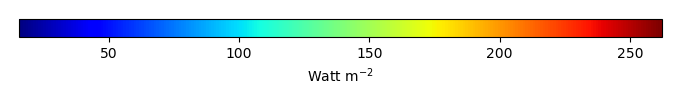MODEL MEANBIAS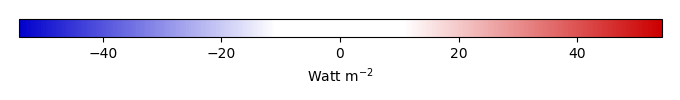BIAS SCORERMSE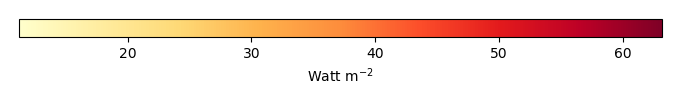RMSE SCOREBENCHMARK INTERANNUAL VARIABILITY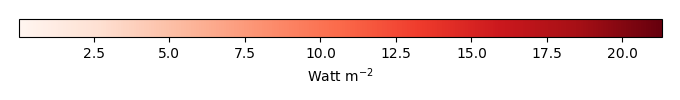MODEL INTERANNUAL VARIABILITYINTERANNUAL VARIABILITY SCOREBENCHMARK MAX MONTHMODEL MAX MONTHDIFFERENCE IN MAX MONTH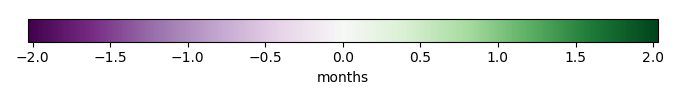SEASONAL CYCLE SCORESPATIAL TAYLOR DIAGRAMMODEL COLORS# Spatially integrated regional mean

MODEL COLORSREGIONAL MEANANNUAL CYCLEMONTHLY ANOMALYANNUAL CYCLE# All Models

BenchmarkCLM4CLM4.5CLM5# Data Information

creation_date: Tue Jul 1 08:29:23 PDT 2014

source_file: This product is generated from monthly 1 degree GEWEX SRB Radiation observations